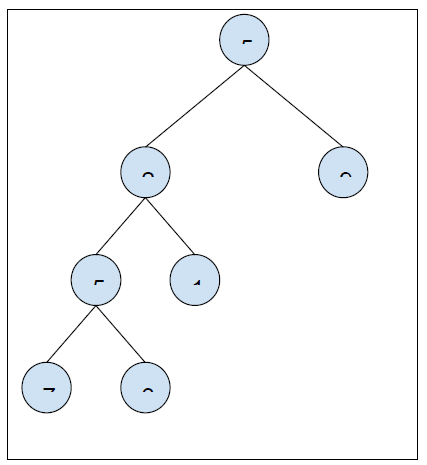# Find Minimum Depth of a Binary Tree in C++

In this problem, we are given a binary tree. Our task is to Find Minimum Depth of a Binary Tree.

Binary Tree has a special condition that each node can have a maximum of two children.

The minimum depth of a binary tree is the shortest path between the root node to any leaf node.

Let’s take an example to understand the problem,

## Input## Output

2

## Solution Approach

The solution to the problem is by traversing the binary tree and counting the heights. This can be done by recursively calling for the child node of the node for each node non-leaf node and return 1 for each leaf node.

Program to illustrate the working of our solution,

## Example

Live Demo

#include<bits/stdc++.h>
using namespace std;
struct Node {
int data;
struct Node* left, *right;
};
int findMinDepthBT(Node *currentNode) {
if (currentNode == NULL)
return 0;
if (currentNode->left == NULL && currentNode->right == NULL)
return 1;
if (!currentNode->left)
return findMinDepthBT(currentNode->right) + 1;
if (!currentNode->right)
return findMinDepthBT(currentNode->left) + 1;
return min(findMinDepthBT(currentNode->left),
findMinDepthBT(currentNode->right)) + 1;
}
Node *newNode(int data) {
Node *temp = new Node;
temp->data = data;
temp->left = temp->right = NULL;
return (temp);
}
int main() {
Node *root = newNode(5);
root->left = newNode(2);
root->right = newNode(9);
root->left->left = newNode(5);
root->left->right = newNode(1);
root->left->left->left = newNode(7);
root->left->left->right = newNode(3);
cout<<"The minimum depth of binary tree is "<<findMinDepthBT(root);
return 0;
}

## Output

The minimum depth of binary tree is 2

This approach is quite efficient but we can use other traversal techniques to find the minimum depths more effectively.

One such approach is using level order traversal in which we traverse the tree level by level. And we will return the level number in which we encounter our first leaf node.

Program to illustrate the working of our solution,

## Example

Live Demo

#include<bits/stdc++.h>
using namespace std;
struct Node {
int data;
struct Node *left, *right;
};
struct lOrderQueue {
Node *node;
int depth;
};
int findMinDepthBT(Node *root) {
if (root == NULL)
return 0;
queue<lOrderQueue> levelOrder;
lOrderQueue deQueue = {root, 1};
levelOrder.push(deQueue);
while (levelOrder.empty() == false) {
deQueue = levelOrder.front();
levelOrder.pop();
Node *node = deQueue.node;
int depth = deQueue.depth;
if (node->left == NULL && node->right == NULL)
return depth;
if (node->left != NULL) {
deQueue.node = node->left;
deQueue.depth = depth + 1;
levelOrder.push(deQueue);
}
if (node->right != NULL) {
deQueue.node = node->right;
deQueue.depth = depth+1;
levelOrder.push(deQueue);
}
}
return 0;
}
Node* newNode(int data) {
Node *temp = new Node;
temp->data = data;
temp->left = temp->right = NULL;
return temp;
}
int main() {
Node *root = newNode(5);
root->left = newNode(2);
root->right = newNode(9);
root->left->left = newNode(5);
root->left->right = newNode(1);
root->left->left->left = newNode(7);
root->left->left->right = newNode(3);
cout<<"The minimum depth of binary tree is "<<findMinDepthBT(root);
return 0;
}

## Output

The minimum depth of binary tree is 2